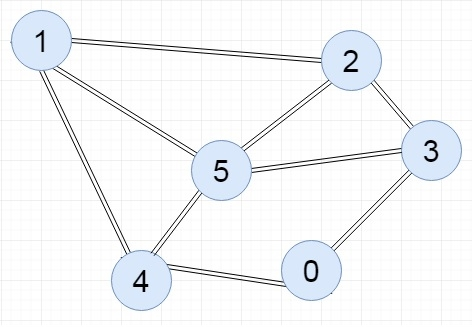# C++ Program to Represent Graph Using Incidence Matrix

The incidence matrix of a graph is another representation of a graph to store into the memory. This matrix is not a square matrix. The order of the incidence matrix is V x E. Where V is the number of vertices and E is the number of edges in the graph.

In each row of this matrix we are placing the vertices, and in each column the edges are placed. In this representation for an edge e {u, v}, it will be marked by 1 for the place u and v of column e.

## The complexity of Adjacency Matrix representation

• The incidence matrix representation takes O(Vx E) amount of space while it is computed. For complete graph the number of edges will be V(V-1)/2. So incidence matrix takes larger space in memory.

InputOutput

E0 E1 E2 E3 E4 E5 E6 E7 E8 1 1 0 0 0 0 0 0 0 0 0 1 1 1 0 0 0 0 0 0 1 0 0 1 1 0 0 0 1 0 0 0 1 0 1 0 1 0 0 1 0 0 0 0 1 0 0 0 0 1 0 1 1 1

## Algorithm

Input − The u and v of an edge {u,v}

Output − Incidence matrix of the graph G

At first, there are edge count ed_cnt is 0 for the incidence matrix.

Begin
ed_cnt := ed_cnt + 1
inc_matrix[u, ed_cnt] := 1
inc_matrix[v, ed_cnt] := 1
End

## Example Code (C++)

Live Demo

#include<iostream>
using namespace std;
int inc_arr; //initial array to hold incidence matrix
int ed_no = 0;
void displayMatrix(int v, int e) {
int i, j;
for(i = 0; i < v; i++) {
for(j = 0; j < e; j++) {
cout << inc_arr[i][j] << " ";
}
cout << endl;
}
}
void add_edge(int u, int v) { //function to add edge into the matrix with edge number
inc_arr[u][ed_no] = 1;
inc_arr[v][ed_no] = 1;
ed_no++; //increase the edge number
}
main(int argc, char* argv[]) {
int v = 6; //there are 6 vertices in the graph
int e = 9; //there are 9 edges in the graph
}
1 1 0 0 0 0 0 0 0
0 0 0 0 1 0 1 1 1